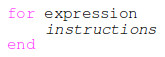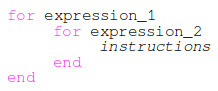# Scilab programming – FOR loops

In this article we are going to learn about:

• simple FOR loops in Scilab
• nested FOR loops in Scilab
• vectorization in Scilab

Before going through this article, it is recommended to have a basic understanding about:

Building complex algorithms often requires repetitive actions. For example we need to evaluate the function f(x) defined below, for different values of x:

$\begin{equation*} \begin{split} f(x) = x^2 + \sqrt(x) \end{split} \end{equation*}$

for x = 1, 2, 3, 4, 5

Without knowing that we can use conditional loops to evaluate the function, the only way to evaluate the function, for every value of x, is to use Scilab console, define x = 1 then evaluate the function, define x = 2 and evaluate the function and so on.

-->x=1;

-->f(x) = x^2 + sqrt(x)
f =

2.

-->x=2;

-->f(x) = x^2 + sqrt(x)
f =

2.
5.4142136

-->x=3;

-->f(x) = x^2 + sqrt(x)
f =

2.
5.4142136
10.732051

-->x=4;

-->f(x) = x^2 + sqrt(x)
f =

2.
5.4142136
10.732051
18.

-->x=5;

-->f(x) = x^2 + sqrt(x)
f =

2.
5.4142136
10.732051
18.
27.236068

-->

In the example above, the function f is a vector and x is function argument and index.

Evaluating function f in the Scilab console, for different values of x is not very efficient and it’s time consuming. A better way to do it is to use a conditional loop. This means that we will evaluate an instruction repeatedly until a condition is satisfied.

A common conditional loop is the for loop. It’s named conditional because it’s executed depending if a conditions is true or false. If the condition is true we execute the instruction, if it’s false we stop executing it.

#### Simple FOR loops

The general syntax of the for loop in Scilab is the following:The expression contains a set of Scilab operations which perform:

• variable initialisation
• variable comparison (condition)
• variable incrementation each time the instruction is executed

The instruction is the piece of code that is evaluated each time the control variable is incremented as long as the condition within the expression is satisfied.

Let’s apply the for loop structure to our function (in a Scilab script):

for x=1:1:5
f(x) = x^2 + sqrt(x);
end

In our example x is the index which gets incremented and also the function argument. The first value which is assigned to x is 1. We evaluate the function f for x=1. After, we increment x with 1 so it becomes 2. We evaluate again the function for x=2. We do the same operations until x=5.

The results are stored inside a vector f. The first value of f, f(1), corresponds to x=1. The last value of f, f(5), corresponds to x=5.

If we run this piece of script and display the result of the f function in the console we’ll get:

-->f
f =

2.
5.4142136
10.732051
18.
27.236068

-->

We have the same result as the previous example but with a more compact code.

Bear in mind that the variable x used in the example above has two roles: as index and argument. We’ll give some other examples in which there is a distinction between the index and the function argument.

In the image below you can see a logical diagram of the for loop, the events that happen during the loop and the corresponding Scilab instructions for each event.

Another example where for loops are very handy is the following. Let’s suppose you need to create a vector t_vec which contains time values, from 0.1 to 10 seconds, in increments of 0.1. If you define the vector by hand, you’ll need to enter 100 values, something like:

-->t_vec = [0.1 0.2 0.3 0.4 0.5 0.6...

This in not efficient at all. By using a for loop the definition of the vector is:

for i=1:100
t_vec(i) = i/10;
end

#### Nested FOR loops

Nested for loops means a for loop inside another for loop. The general syntax for nested for loops is:Let’s think for an example in which we can use nested for loops. What about a way to generate a matrix? For example we need a matrix s_mat, with string elements, in which each element is representing the line number and column number (e.g. ’11’, ’12’, ’13’, …). The matrix should be 5 x 5.

for i=1:5
for j=1:5
s_mat(i,j)=string(i)+string(j);
end
end

By evaluating the above instructions inside a Scilab script and typing s_mat + <Enter> at the console, we get:

-->s_mat
s_mat =

!11 12 13 14 15 !
!               !
!21 22 23 24 25 !
!               !
!31 32 33 34 35 !
!               !
!41 42 43 44 45 !
!               !
!51 52 53 54 55 !

-->

#### Trigonometric function evaluation

Let’s have a look on another example for for loops. We have the trigonometric circle and we want to calculate the sinh (hyperbolic sine) multiplied with cosh (hyperbolic cosine) function for multiple angles, starting from 0°, incremented by π/8, up to 2π.

The definitions of the function to be calculated is:

$\begin{equation*} \begin{split} f(x) =& sinh(x) + cosh(x) \end{split} \end{equation*}$

The Scilab script to evaluate the functions is:

angle = [0:%pi/8:2*%pi];

for i = 1:length(angle)
f(i) = sinh(angle(i)) * cosh(angle(i));
end

In this example the argument of the function alpha is different than the index i.

The angle variable is defined as a vector, starting from 0 up to 2π, with π/8 increments. The i is the index of the angle vector, and f is the function we want to calculate.. The loop is executed, starting with index 1, for each value of the angle vector. Using the Scilab function length we extract the number of elements of the vector (in our case is 17).

#### Vectorization (array programming)

For these simple example we can also use vectorization also named array programming. Vectorization means that we apply mathematical operations to array (vector) variables instead of scalars (individual elements).

For our particular example we can calculate the f function simply as:

-->x = [1 2 3 4 5];

-->f = x.^2 + sqrt(x)
f =

2. 5.4142136 10.732051 18. 27.236068

-->

Conditional loops are heavily used in any kind of programming languages. Keep practising for different examples and you’ll naturally start to use conditional loops where repetitive calculations are required.

For any questions, observations and queries regarding the article, use the comment form below.

Don’t forget to Like, Share and Subscribe!

#### Ad Blocker Detected

Dear user, Our website provides free and high quality content by displaying ads to our visitors. Please support us by disabling your Ad blocker for our site. Thank you!